X

## Triangle Calculator - SSS

Requires all three sides of triangle.

## Triangle Calculator - SAS

Requires two sides and one included angle.

## Triangle Calculator - SSA

Requires two sides and a non-included angle.

## Triangle Calculator - ASA

Requires one side of the triangle and two adjacent angles.

## Triangle Calculator - AAS

Requires two angles and one opposite side.

## Triangle Calculator - Equilateral

Requires side length of the equilateral triangle.

## Triangle Calculator - Right Angle

Requires two side lengths of a right angle triangle.

Remember, the input can only be in feet (ft), inches (in), yards (yd), centimetres (cm), millimetres (mm) and metres (m) but never a combination of two different units!

Area of a Triangle = (1/4) x √ [ (a+b+c) x (b+c-a) x (c+a-b) x (a+b-c) ]
Price per unit (drop down ft2, in2, yd2, cm2, mm2, m2)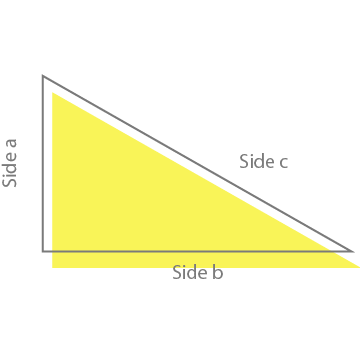### What is a triangle?

A triangle is a special closed shape or a polygon that has three vertices, three sides and three angles. A vertex is a point where two lines or sides meet. Since a triangle has three sides, it also has three vertices a, b and c. The total of interior angles, is always 180°. A triangle can be classified based on their side length and interior angles.

For example, a triangle with equal side lengths can be identified as equilateral triangle or a triangle none of the lines / sides having same length is scalene triangle.

#### Triangles identified by side lengths: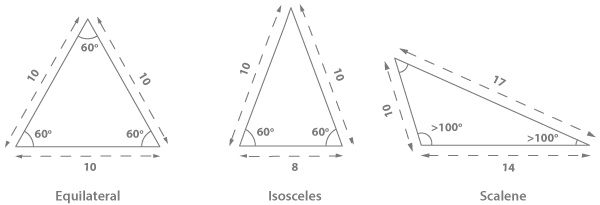#### Triangles identified by angles: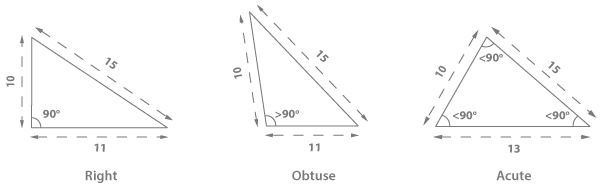### Types of triangle:

Triangles can be classified as 6 different types, based on their side length and angles.

1. #### Acute angled triangle:

A triangle where all three angles are less than 90°.
∠ABC, ∠ACB and ∠BAC are all acute angles.

2. #### Right angled Triangle:

A triangle which has one angle of 90°.
∠ABC = one right angle.

3. #### Obtuse angled Triangle:

In obtuse triangle, any one of the triangles is greater than 90°.
∠ABC

4. #### Equilateral Triangle:

When all side lengths of a triangle, are equal. It’s called equilateral.
Here AB = BC = CA.

5. #### Isosceles Triangle:

A triangle with at least two sides of equal length is Isosceles triangle.
Here AB = AC.

6. #### Scalene Triangle:

A triangle where all sides are of different length.

### Triangle Facts:

A triangle cannot have more than one sides greater than or equal to 90°. As mentioned above in triangle definition, a triangle is a closed path.

The sum of all internal triangles is always 180°.

The total of any two side lengths is always greater than the third side length.

### Pythagorean theorem:

Pythagorean theorem, is a theorem about right triangle. According to Pythagorean theorem, a square of the length on hypotenuse side (longest side) is equal to the total of two other sides. Any triangle that satisfies this condition is a right angled triangle.

Pythagorean Equation:

a2 + b2 = c2
EX: Given a = 5, c = 7, find b:
52 + b2 = 72
25 + b2 = 49
b2 = 24 => b = 4.8989

### Law of sines:

According to law of sines, the ratio between the length of a side and the sine of its opposite angle is constant. Law of sines helps finding any missing length or angle of a triangle. For example: if side length of a and A and B angles are known. We can easily find the side length of b by plugging the given information in the formulae below:

In case where all side lengths are known, angles of the triangles can be calculated as follows:### How to calculate the area of a triangle?

There are many methods to calculate the area of a triangle. Choosing a method depends on the information available. Most common method to find out the area of a triangle is:In case where all side lengths are known, angles of the triangles can be calculated as follows:In a scenario, where two sides and an angle is given. The slight change in formula can be done to get the area of a triangle. The formula will be:There is one more method of calculating area of a triangle using Heron’s Formula which requires the all three sides to be known:### Median:

Median of a triangle is the length of a line that is drawn from the vertex of a triangle to the midpoint of the opposite side. A triangle has three medians which intersect each other at centroid of triangle.

A centroid is arithmetic mean position of all the points in triangle.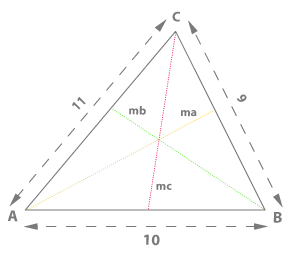Ma = Median of side a
Mb = Median of side b
Mc = Median of side c
Median of each side can be calculated as follows:The inradius is the radius of a circle drawn inside a triangle which touches all three sides of a triangle i.e. inscribed circle. The center of this circle is the point where two angle bisectors intersect each other. It’s perpendicular to any of the three sides of triangle.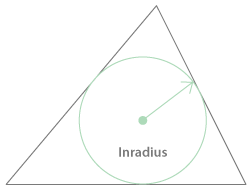Where s = a + b + c / 2
Where a, b and c are the side lengths of the triangle.

In case of triangle, the circumradius is the radius of a circle that passes through all the vertices of a triangle. The center point of this circle is called circumcenter. Circumcenter is a point where all perpendicular bisectors of each side of a triangle meet each other.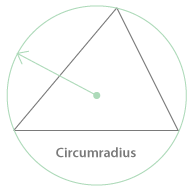Circumradius = a / 2 * sin(A)
Where a is a side length and A is the angle opposite side a.
Although side a and angle A are being used, any of the sides and their respective opposite angles can be used in the formula.

# Understanding the Square footage:

To understand how to calculate square footage we must first begin with the definition of area. An area is the size of a two-dimensional surface. The area of a triangle is the space contained within its 3 sides. To find out the area of a triangle, we need to know the length of its three sides. The sides should be measured in feet (ft) for square footage calculations and if needed, converted to inches (in), yards (yd), centimetres (cm), millimetres (mm) and metres (m).

The formula:
Area of a Triangle = (1/4) x √ [ (a+b+c) x (b+c-a) x (c+a-b) x (a+b-c) ]
Length of side a (drop down ft, in, yd, cm, mm, m)
Length of side b (drop down ft, in, yd, cm, mm, m)
Length of side c (drop down ft, in, yd, cm, mm, m)
Answer = ((1/4) x √ [ (a+b+c) x (b+c-a) x (c+a-b) x (a+b-c) ])
Abbreviations of unit area: ft2, in2, yd2, cm2, mm2, m2

Where do you need it in daily life?

Our Triangle Calculator helps you calculate the area required for a triangle shape. Although we cover most common use case e.g. You may know two sides and an included angle but would like to know the missing side length. we have also recently added Right triangle calculator which also a commonly used in a scenario where you know two side lengths of a triangle one of which is 90° Deg.

What measurements do you need?

You need to know the length of the three sides of the triangle in either feet (ft), inches (in), yards (yd), centimetres (cm), millimetres (mm) or metres (m).

What can you calculate with this tool?

You can calculate the area of the triangle in square feet, in square inches, square yards, square centimetres, square millimetres and square meters. Yes, our tool is that awesome.

Our calculator gives you the option of calculating the exact cost of materials. All you have to do is enter the price per unit area and voila, you have the total cost of materials in a single click!

Conversion factors:

To convert among square feet, square inches, square yards, square centimetres, square millimetres and square meters you can utilize the following conversion table.

 Square feet to square yards multiply ft2 by 0.11111 to get yd2 Square feet to square meters multiply ft2 by 0.092903 to get m2 Square yards to square feet multiply yd2 by 9 to get ft2 Square yards to square meters multiply yd2 by 0.836127 to get m2 Square meters to square feet multiply m2 by 10.7639 to get ft2 Square meters to square yards multiply m2 by 1.19599 to get yd2 Square meters to square millimetres multiply the m2 value by 1000000 to get mm2 Square meters to square centimetres multiply the m2 value by 10 000 to get cm2 Square centimetres to square metres multiply the cm2 value by 0.0001 to get mm2 Square centimetres to square millimetres multiply the cm2 value by 100 to get mm2 Square millimetres to square centimetres multiply the mm2 value by 0.000001 to get cm2 Square millimetres to square metres multiply the mm2 value by 1000000 to get m2